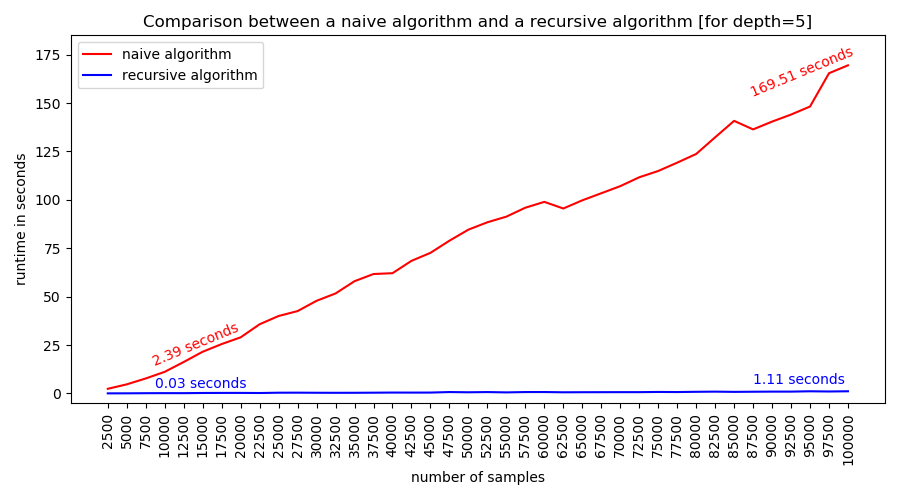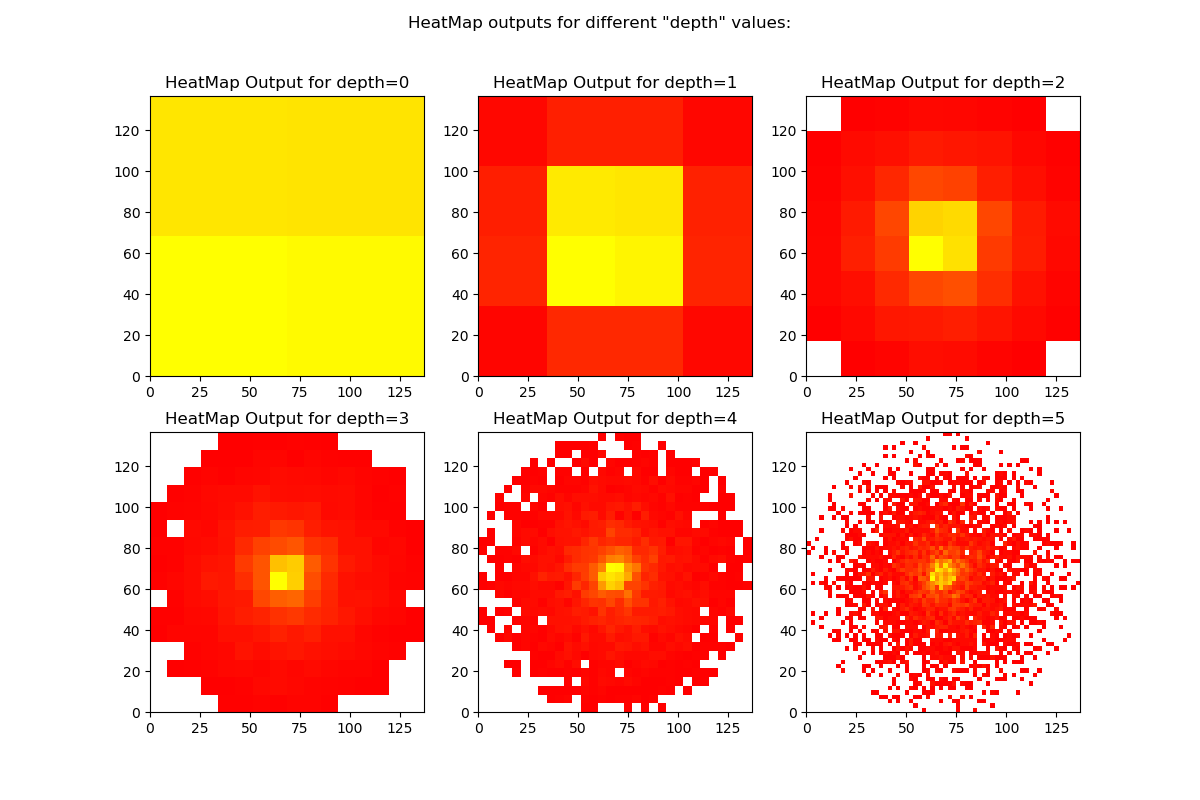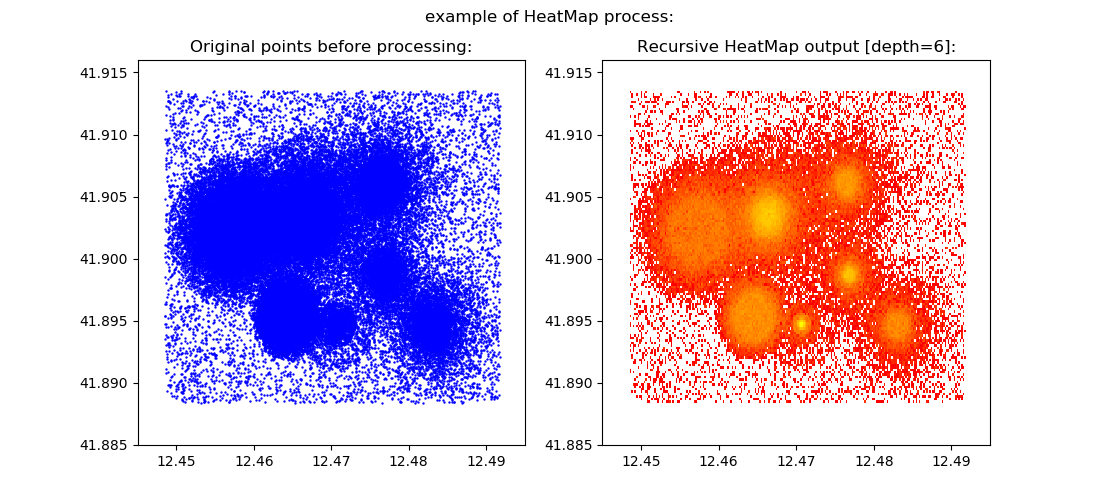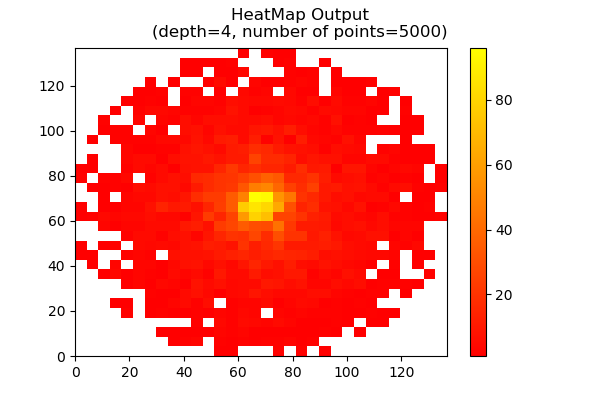# Recursive Heatmap Calculation

recursive method for heatmap calculation from given points.
Alternatives To Recursive Heatmap Calculation
Gazepointheatmap40
5 years agogpl-3.0Python
Easy to use Python command line based tool to generate a gaze point heatmap from a csv file. 👁️
Csv Heatmap33
8 years ago1mitHTML
Dump1090.socket3000320
6 years ago3gpl-3.0Perl
collect dump1090 flight positions using socket30003 and save them as csv
Stravaheatmap18
6 years agoPython
Make a simple heatmap from your strava data
Geo Heatmap7
4 years ago1mitPython
Draws geographical heatmap from csv file
Cumulative Heatmap Calculation4
2 years agomitPython
fast calculation of heatmap from given points.
Muvias3
5 years agootherJavaScript
Regionalcorrelation3
3 years agoPython
Telematics Heatmap3
3 years agoJavaScript
A heatmap visualization library for sighting intensity of data at geographical points contained in generic CSV files
Csv Data Heat Map3
2 months ago1gpl-3.0Python
CSV Data to Heat Map Converter
Alternatives To Recursive Heatmap Calculation
Select To Compare

Alternative Project Comparisons

# Recursive-HeatMap-Calculation

recursive method for heatmap calculation from given 2D points.

## Overview

Calculating a heat map is a complex task, because since the user selects a more detailed resolution, the runtime of the calculation increases accordingly. The main difficulty in the calculation is in the sum of all the coordinates for the boundaries of each cell in the heat map. To overcome this problem, the code smart_heatmap.py applied recursive solution:

Note: In one of my other projects, there is a method with even faster runtime! see: cumulative-heatmap-calculation

• Step One: Initialize a small amount of low-resolution square cells.
• Step Two: For each of the squares we will isolate only the coordinates that within to its boundaries and sum them.
• Step Three: Now, split each square into four small squares in equal size. We will perform Step Two for each of these new squares, but now it will only be performed on the coordinates that matched the boundaries of the original square.
• Step Four: Repeat the process recursively until we reach squares size of the desired resolution.

If we encounter during the process a square that does not intersect at all with the coordinates, we will stop its division. Now, we will save all the squares we calculated with intersection count bigger than zero. Now we got a heat map with the required resolution! As can be seen, the runtime of the calculation using the recursive algorithm is significantly lower than the naive algorithm:The resolution of the heat map can be adjusted using the "depth" variable. This variable determines how many splits to make in each of the first squares. The larger the "depth" variable, the higher the resolution we get. You can see a diagram describing the split into squares, by each depth:As mentioned, it is important to make sure that a suitable resolution is chosen for the calculation, since different values for the "depth" variable will lead to different results. A simple example based on the file circle.csv illustrates how different values resulted different outputs:The heat map calculation performed using the HeatMap object. This object receives a Python list consisting of tuples of X and Y coordinates. Also, the desired depth also must be set for the object. Using the data about the coordinates, the HeatMap calculates the initial squares and performs the recursive calculation on them. At the end of the process, the squares that compose the heat map are calculated, as can be seen in the example process figure (based on the file rome_100000.shp that contain 100,000 points coordinates!):The final squares can be accessed as one of the data of the object: HeatMap(xy_tpl_lst, depth = 5).heatmap. It is also possible to create a plot based on the results of the heat map using the function built into the object, as in the following example:

``````from smart_heatmap import HeatMap, loadcsv
hm = HeatMap(xy, depth=4)
hm.plot()
``````In addition, the calculation results can be exported and saved as files in various formats using the save_map function of the object, as can be seen in the implementation.py file. The function allows to save the results in SHP, KML and CSV format. It should be noted that saving the results as layers is automatically set to geographic coordinate system of WGS_1984_DD.

Also, the data that saved to a KML file gets a color corresponding to the count of its intersection. Example for such output you can see here as interactive MYMAPS page. This output based on the Gibraltar.kml example file and the output also available here: Gibraltar_Output.kml. Also, for convenience, the code contains three XY-tuple-list load functions from SHP, KML and CSV files:

• loadcsv (this function required also the X & Y fields names)

## Libraries

The code uses the following libraries in Python:

matplotlib

shapefile

simplekml

pandas

numpy

## Application

An application of the code is attached to this page under the name:

implementation.py

the examples outputs are also attached here.

## Example for using the code

To use this code, you just need to import it as follows:

``````# import

# define depth variable
depth = 5

# application
hm = HeatMap(xy, depth)

# plot
hm.plot()

# save data as csv
hm.save_map('filename','csv')
``````

When the variables displayed are:

xy: the given xy coordiantes list.

depth the required depth for the recursion, define the output resolution.